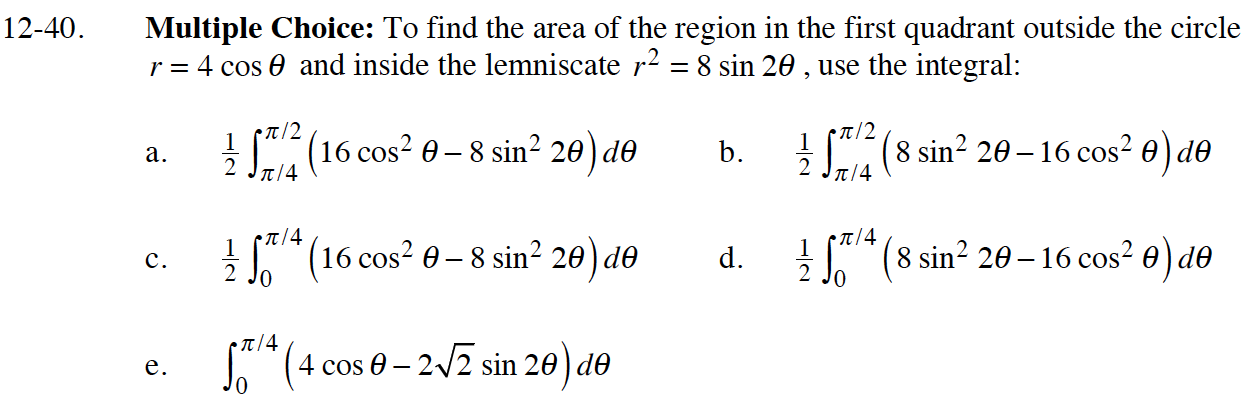### Home > CALC > Chapter Ch12 > Lesson 12.1.3 > Problem12-40

12-40.Determine where the functions intersect for the bounds of integration. Remember, this is only in the first quadrant.

$16\cos^2(\theta)=8\sin(2\theta)$

$16\cos^2(\theta)=16\sin(\theta)\cos(\theta)$

Graph the functions to determine which is the "upper" function and which is the "lower" function.

Use the area inside polar curves formula: